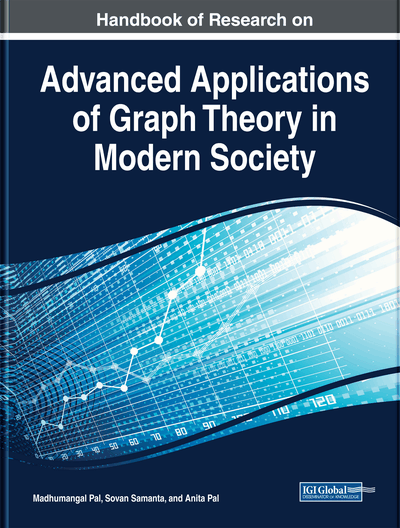# Graph Theory: Novel Multiple-Attribute Decision-Making Effect

Seethalakshmi R. (SASTRA University (Deemed), India)
DOI: 10.4018/978-1-5225-9380-5.ch014
Available
\$37.50
No Current Special Offers

## Abstract

Mathematics acts an important and essential need in different fields. One of the significant roles in mathematics is played by graph theory that is used in structural models and innovative methods, models in various disciplines for better strategic decisions. In mathematics, graph theory is the study through graphs by which the structural relationship studied with a pair wise relationship between different objects. The different types of network theory or models or model of the network are called graphs. These graphs do not form a part of analytical geometry, but they are called graph theory, which is points connected by lines. The various concepts of graph theory have varied applications in diverse fields. The chapter will deal with graph theory and its application in various financial market decisions. The topological properties of the network of stocks will provide a deeper understanding and a good conclusion to the market structure and connectivity. The chapter is very useful for academicians, market researchers, financial analysts, and economists.
Chapter Preview
Top

## Introduction

### Graph Theory – Relevant to Mathematics

Mathematics acts a critical and essential need in different fields. One of the significant roles in Mathematics is played by Graph theory of structural models. The structural arrangement paves the way for innovative methods models in various disciplines for better strategic decisions

In mathematics, graph theory is the study through graphs by which the structural relationship studied with a pairwise relationship between different objects. A graph consists of lines or arcs connected by nodal points or vertices which are also connected edges. The different types of network theory or models or model of the network are called as graphs. These graphs do not form a part of analytical geometry, but they called as graph theory which is ‘ points connected by lines.’

### Elements of Graph Theory

A pair that has set of nodes V(G) and a set of edges E(G) is called a graph, represented by G = (V, E). The adjacency relation of a graph G is a symmetric binary relation induced by the edge set E(G) on a vertex set V(G). Dual nodes i and j are said to be adjacent when there exists an edge between i and j.

### Degree

The number of edges incident on a node ‘i' is representing the amount of that node and is named by ‘di.’ There are two types of graph namely ‘Direct graph’ and ‘Indirect graph.’ The ‘in degree, di-’ and ‘out degree, di+,’ of node i are to be distinguished in the direct graph.

#### Directed and Undirected Graph

The nodes in an undirected graph are connected by bidirectional edges. On the other hand, the nodes in a direct graph are connected by edges that point in one direction.

### Regular Graph

A regular graph consists of nodes all of which are of the same degree. For example, all nodes in of ‘k-regular’ graph are of degree ‘k.’

The adjacency matrix, A(G) in the graph G, is a square matrix of order n in which the entry aij is 1if the edge eij∈ E(G), otherwise aj is 0. The matrix Ais symmetric, i.e.ai j= aji∀i,j∈ V(G) for an undirected graph.

### Path

A sequence of edges and nodes which are connected in such a way that all nodes are distinct from each other. For example, Pk denotes a path with ‘k’ nodes. The number of edges in a path is said to be the path length and is represented by d(u, v).

### Diameter

The utmost or maximum of d(u,v) over all nodes u and v is said to be the diameter of a graph.

### Walk

The list {v0, e01, v1,...,vk−1, ek−1k, vk}, of alternating edges and nodes is a walk.

### Trail

The no repeated edge with the walk is known as a trail and with no repetitive node is a path.

### Geodesic Distance

The shortest path distance between the two nodes are called as Geodesic distance.

### Circuit

A closed trail is called as a circuit. In a circuit, the endpoints of a trail are the same.

### Cycle

If a circuit has no repeated node, then it is called a cycle. A cycle on n nodes is denoted by Cn. A cycle can be a circuit whereas a cycle need not be a circuit.

## Complete Chapter List

Search this Book:
Reset